# Linked List Traversal

Problem Statement: Write a Program for Linked List Traversal or traversing a singly linked list.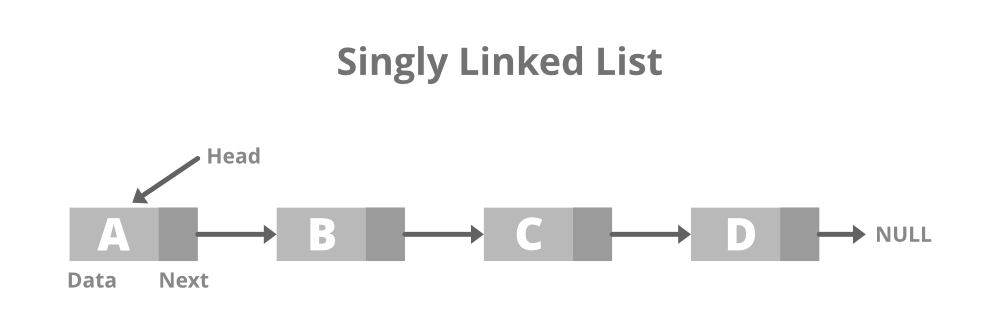Examples:

```Example 1:
Input: N = 5, List = {1, 2, 3, 4, 5}
Output: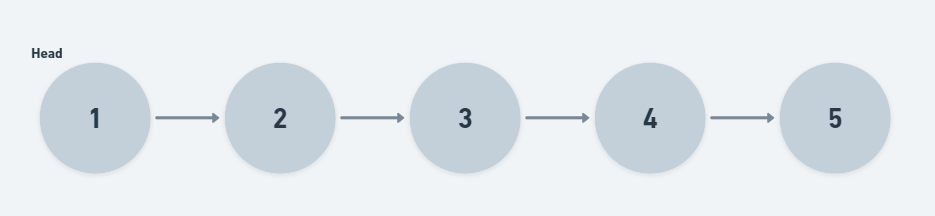```
```Example 2:
Input: N = 1, List = {1}
Output: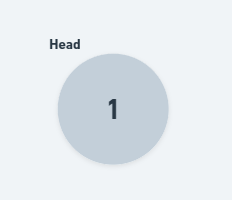```

### Solution:

Disclaimer: Don’t jump directly to the solution, try it out yourself first.

### Approach:

The main idea is to make a curr pointer and initially make it point to the head. Now start iterating the linked list. While the curr pointer does not become NULL, move it forward and print the value of the curr node.

The algorithm walkthrough is shown below:

Suppose the linked list is: {1 -> 2 -> 3 -> 4 -> 5}

Step 1: Make curr points to the Head of the linked list.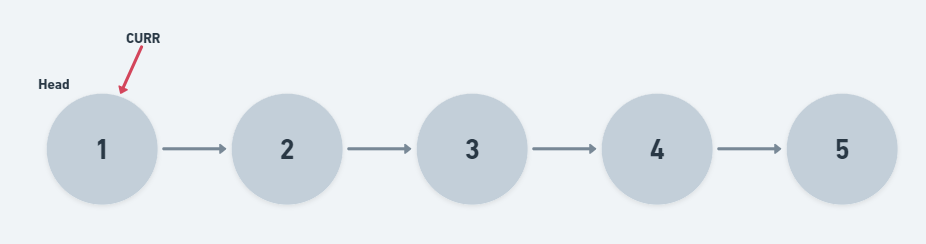o/p => {1} // Output is head->val for now

Step 2: Now iterate over the linked list, till curr != NULL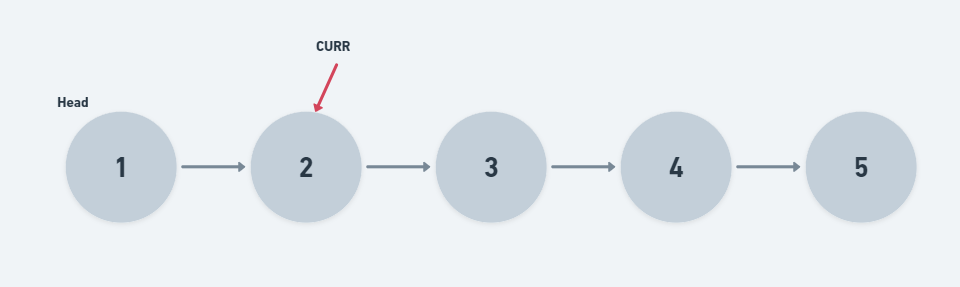o/p => {1, 2}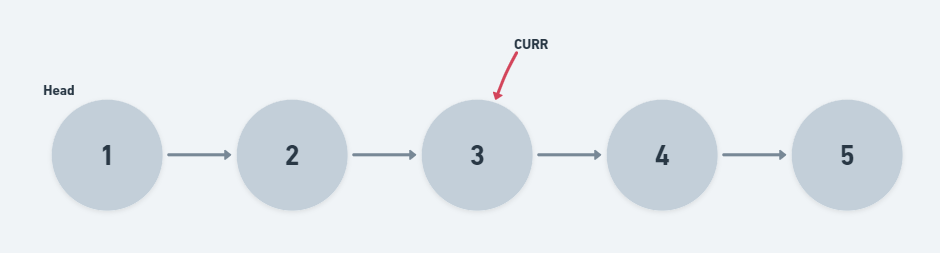o/p => {1, 2, 3}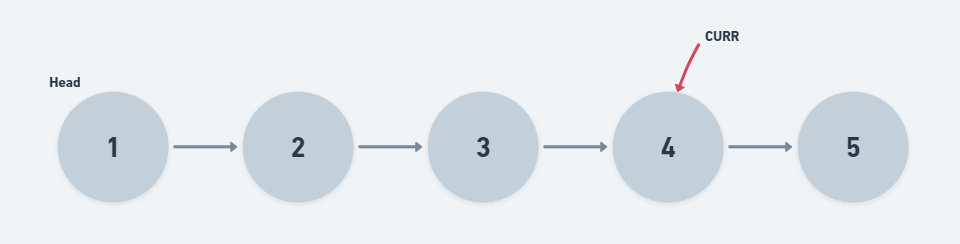o/p => {1, 2, 3, 4}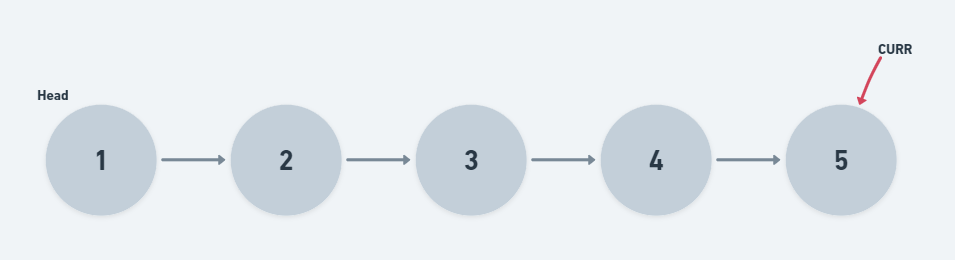o/p => {1, 2, 3, 4, 5}

Step 3: (curr == NULL), Now the Linked List traversal is completed.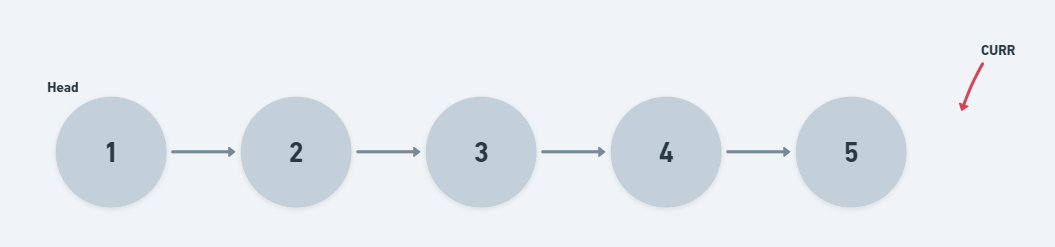Code:

## C++ Code

``````void LinkedListTraversal(ListNode* head) {
cout<<"List is Empty..."<<endl;
}
while(curr != NULL){
cout<<curr->val<<" ";
curr = curr->next;
}
}
``````

Time Complexity for Traversing Linked List: O(N), where N is the number of nodes

Space Complexity for Traversing Linked List: O(1)

## Java Code

``````public void LinkedListTraversal(ListNode head) {
System.out.println("List is Empty...");
}
ListNode curr = head;
while(curr != null){
System.out.print(curr.val + " ");
curr = curr.next;
}
}
``````

Time Complexity for Traversing Linked List: O(N), where N is the number of nodes

Space Complexity for Traversing Linked List: O(1)

Special thanks to Sagar Srivastava for contributing to this article on takeUforward. If you also wish to share your knowledge with the takeUforward fam, please check out this article